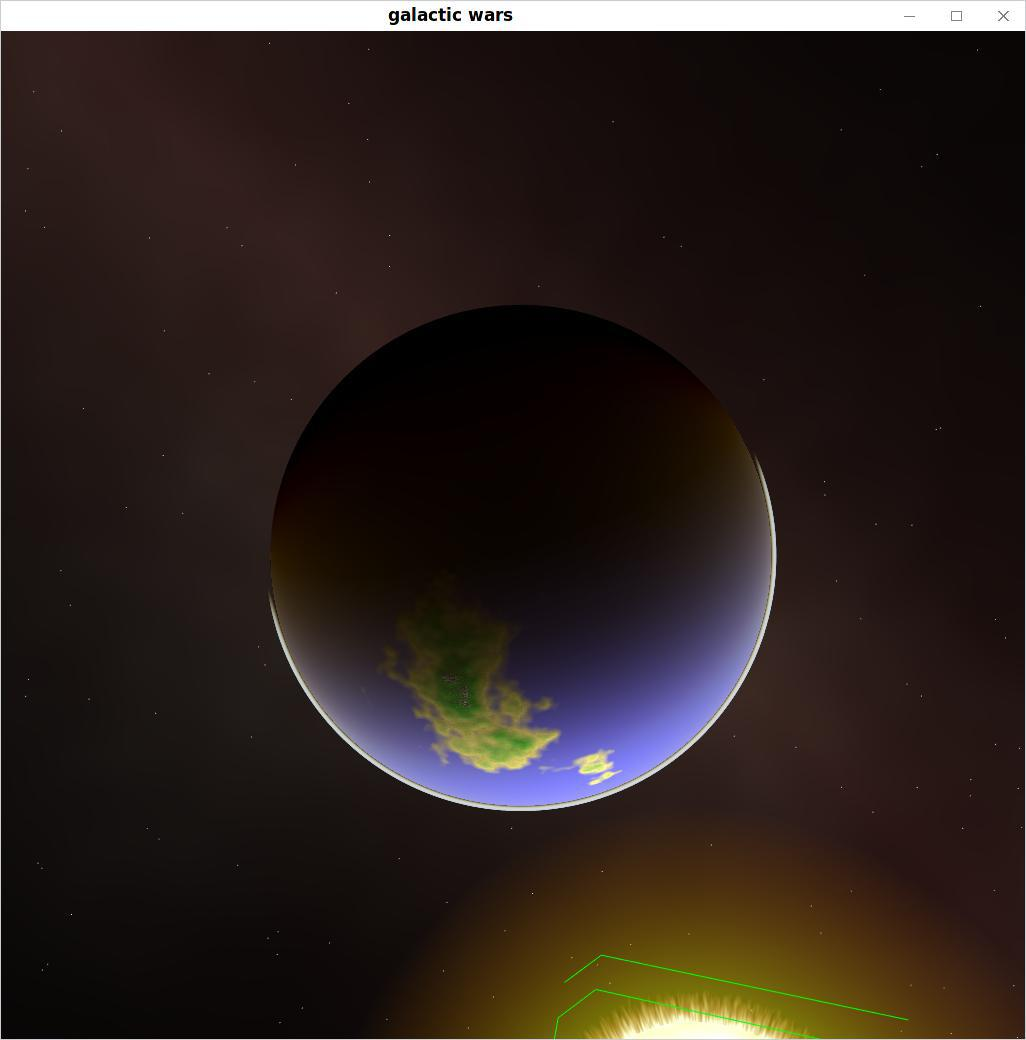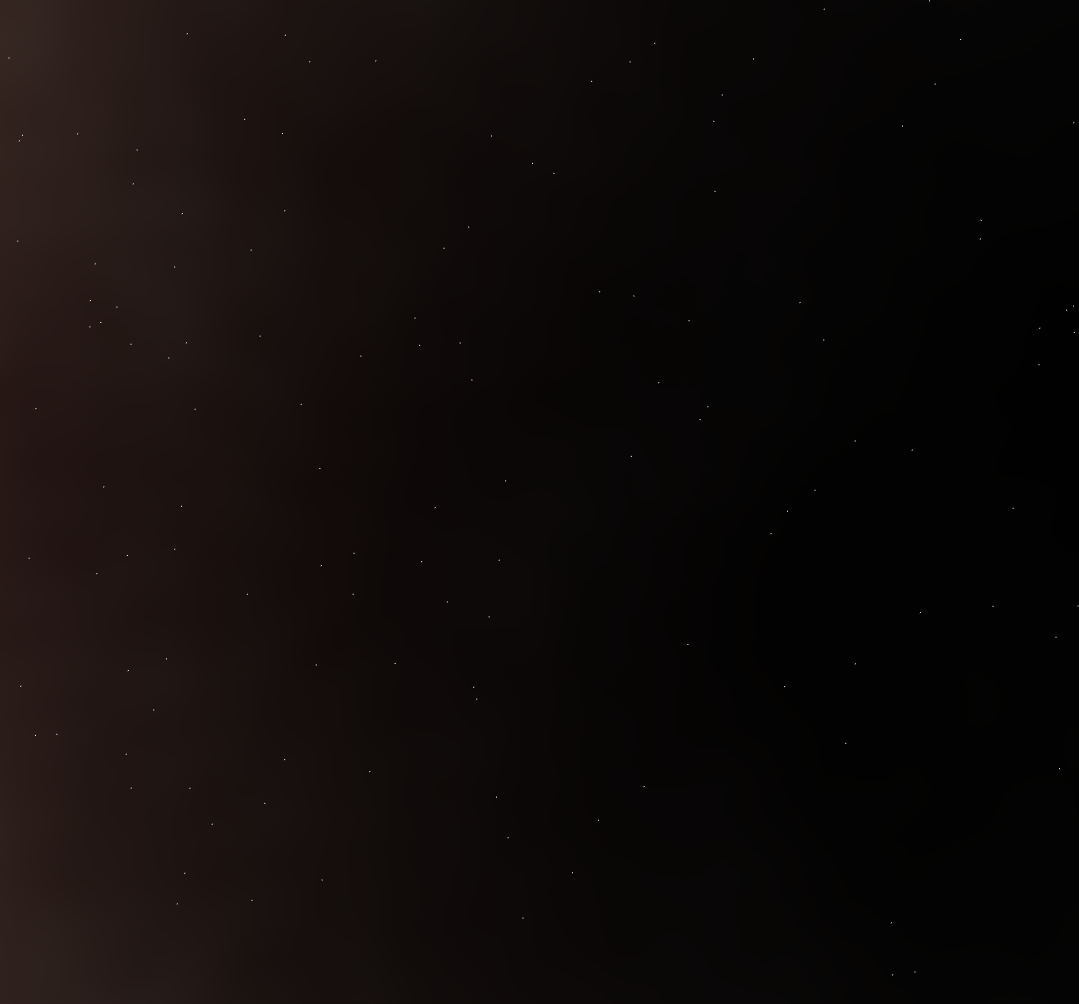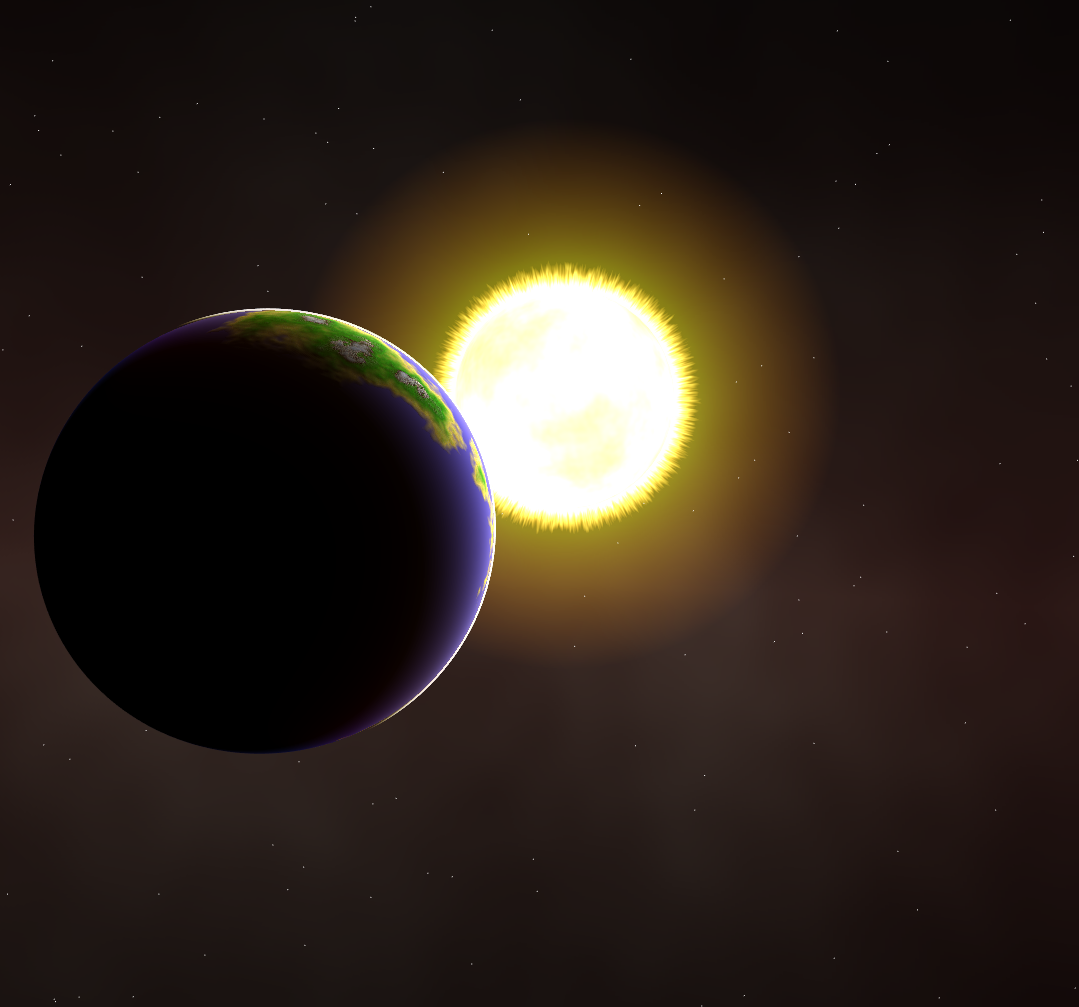I planned to create a 3D strategy game in space with many procedurally generated graphism to avoid loosing time in creating artworks. Here are some tricks I did.
Space
To generate the space, I use a 3D perlin noise in a cubemap. For each pixel, the value of the noise is mixed with different coefficients to generate the 3 components R, G, B of the color. Then the result is multiply by a factor which is inversely proportional to the distance to a virtual plane of direction $\vec{n}$. As the plane is centered, and the maximal distance is 1 because a point is represented by a direction vector in a cubemap, the distance is just the absolute value of the dot product $\vec{n} \cdot \vec{v}$ and the coefficient is $(1.0 - \vec{n} \cdot \vec{v})^2$. Then stars are added by drawing a generated clouds of GL_POINT.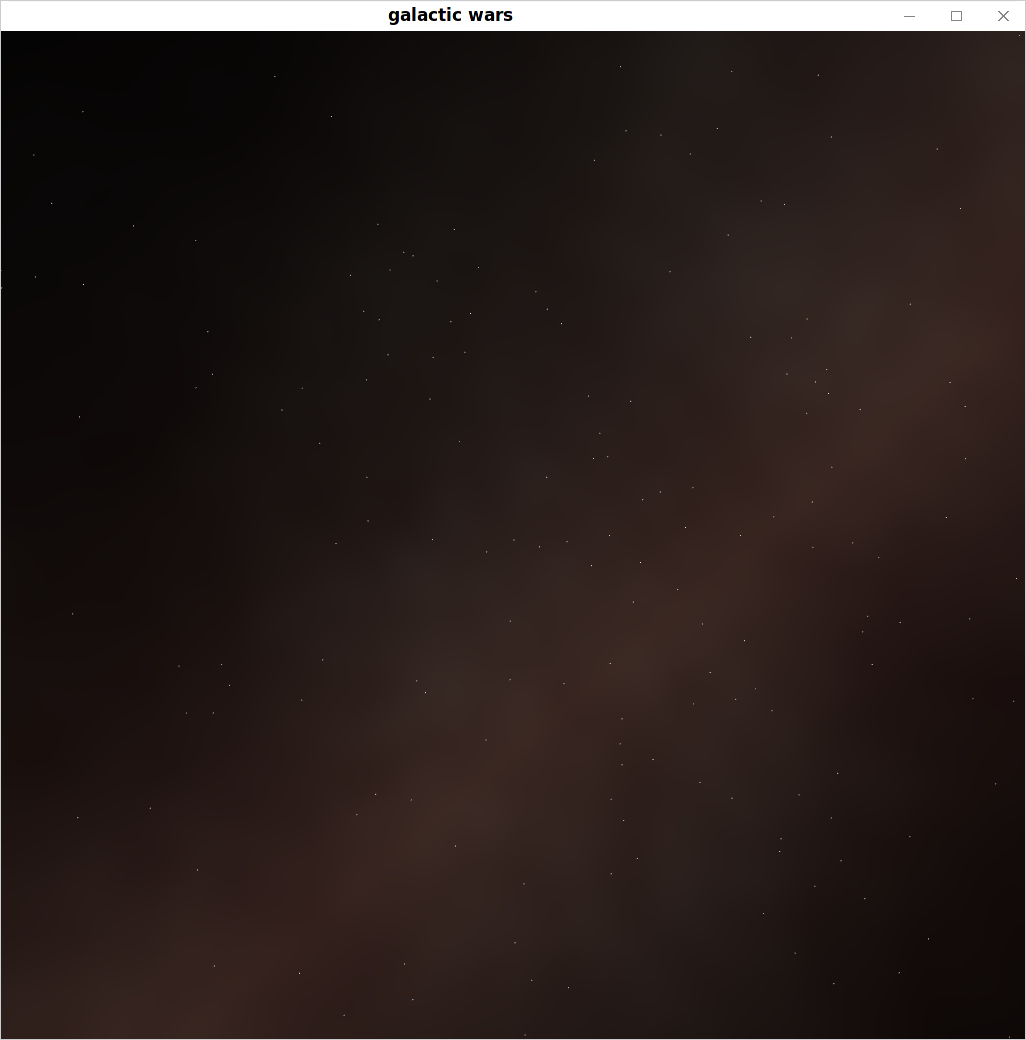Sun
To make the sun I used 3 shaders. One for the gas cloud around the sun. One for the "corona" of the star and one which simply render the star itself with the color mixed with a precomputed 3D perlin noise on a cubemap.
Gas cloud
The gas cloud is a plane which always front the camera. This is done by creating an orthonormal basis with the vector from the plane center to the camera position and create the rotation matrix of the plane from that basis. The pixel color in the gas cloud is a fixed color vector divided by its distance to the center of the plane $l$ and the alpha component is $1.0 - l$ because then length from the center to the nearest border is 1.0 and alpha should be 0 at the border.
Corona
The corona is a half sphere always facing the camera. A 2D perlin noise is generated in realtime with the projected position x, y on the plane facing the half sphere. Then color yellow and red are mixed according to the noise value then mixed again with white according to a function of the noise and the distance in the sphere the ray from the camera goes through. The blending value alpha is dependant on the same function. Each parameters are then tricked to give good results. The length of a ray through a sphere can be computed by solving the following equation: $$x^2 + y^2 + z^2 - r^2 = 0$$ where $x, y, z$ are the component of the vector $\vec{o} + \vec{v} \cdot \lambda$ where $\vec{o}$ is the origin, $\vec{v}$ the direction vector of the ray and $\lambda \in \R$ and $r^2$ is the squared radius of the sphere. We have then $$(\vec{o}.x + \lambda \vec{v}.x)^2 + (\vec{o}.y + \lambda \vec{v}.y)^2 + (\vec{o}.z + \lambda \vec{v}.z)^2 - r^2 = 0$$ After distribution we have that $$\vec{o} \cdot \vec{o} + \lambda^2(\vec{v}\cdot\vec{v}) + 2 \lambda(\vec{o} \cdot \vec{v}) - r^2 = 0$$ This equation has two solutions which once replaced in our vector $\vec{o} + \lambda \vec{v}$ gives us the two intersections with the sphere. The idea to use a hemisphere instead of a plane is that is looks better when we are closer.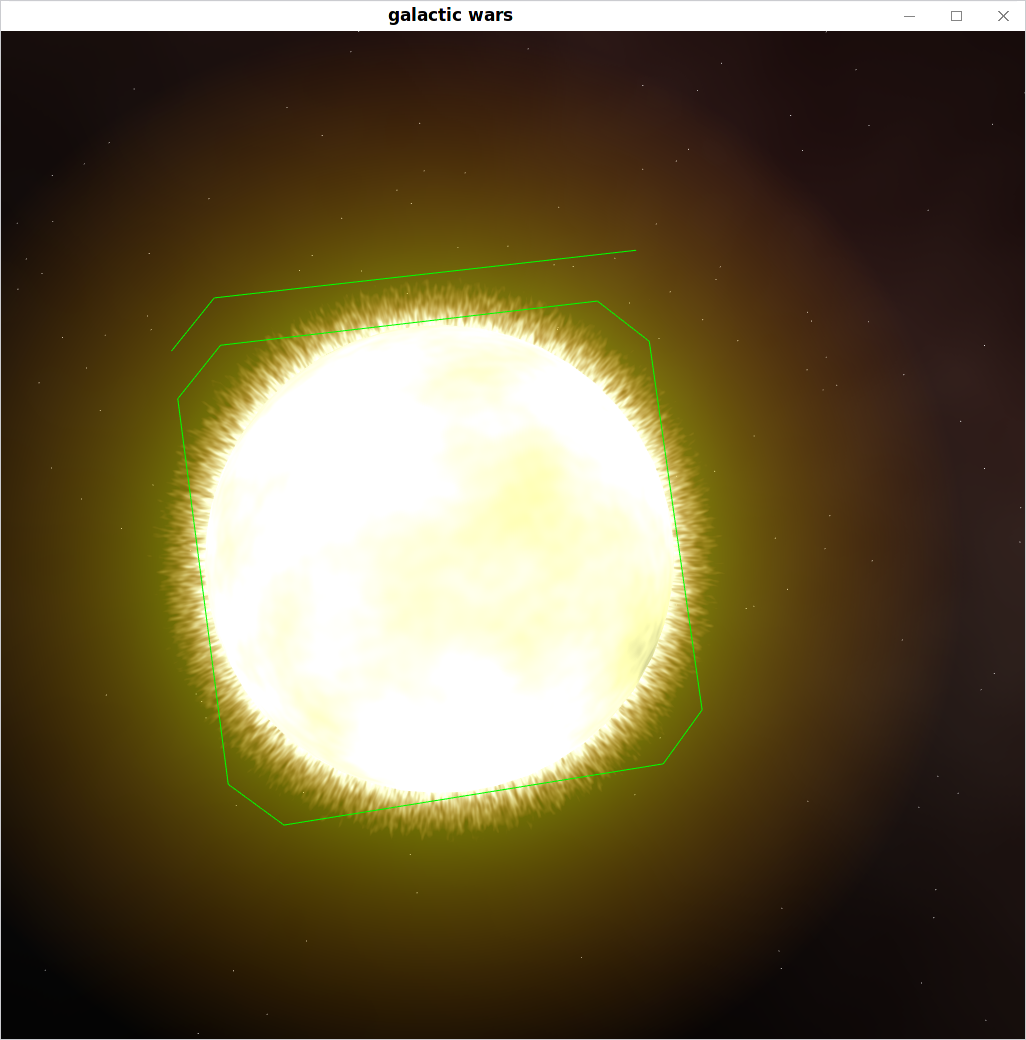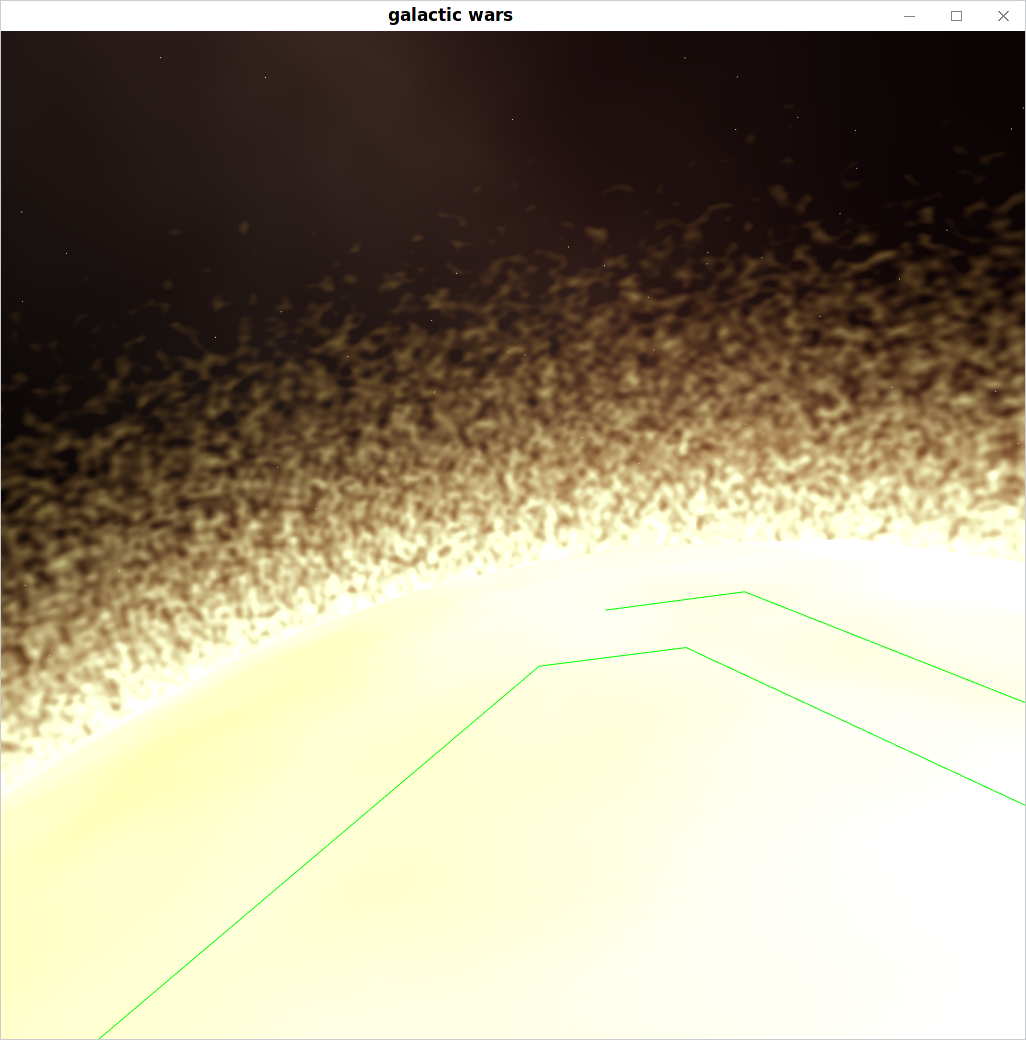Planet
The planet is created from an icosphere where each vertices are displaced according to a 3D perlon noise in a cubemap. The normals are computed by taking an orthogonal basis for the current pixel and then by taking the finite difference on two orthogonal axis of rotation. As the texture is a cubemap, a single face cover $\frac{1}{4}$ of the complete circle so $\frac{1}{4} \cdot 2 \pi$. The angle of rotation to compute the finite difference is then $\frac{\pi}{2} \cdot \frac{1}{texture size}$ The water is just a blue sphere with blending according to the deph of the water. The deph is computed by taking the difference in height between the blue sphere radius and the height of the ground (computed with the perlin noise). The atmosphere is computed using the integral presented in GPU Gem's article http://http.developer.nvidia.com/GPUGems2/gpugems2_chapter16.html. Actually it doesn't look really good but I didn't have the time to find the best parameters. The atmospheric scattering is the result of the following integral: $$\int_A^B e^{\frac{-h}{H_0}} e^{4\pi k(\lambda) \int_u^v e^{\frac{h}{H_0}}ds}$$ Where $A$ and $B$ are the point of intersection of the sphere, $u$ a point on the ray between $A$ and $B$ and $v$ the intersection of the ray from $u$ in the direction of the sun with the outer atmosphere. $e^{\frac{-h}{H_0}}$ is the density of the atmosphere according to the height from the ground.